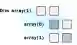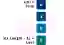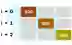# VB.NET Array Examples, String Arrays

Create string and integer arrays with initializers. Get elements, and use Length and For Each loops.

Array. Stones line the road, one after another. In an array, one element is stored after another in a linear way. And like stones, arrays are a foundation.Concepts. Other collections are built with internal arrays. There are many ways to initialize arrays. We can use Array class methods to change them.ToArray

String array. A string array is created in various ways. In the VB.NET language we can create the array with all its data in an initialization statement.
Version 1: The first array is created with an initialization statement. We do not need to specify the size of the array on the left side.
Version 2: The next array uses the longer syntax. You must specify the capacity of the array in the Dim statement.
Info: We loop over the arrays with For Each. And we pass the arrays to a Sub M, which prints their Lengths.
For Each, ForVB.NET program that uses arrays Module Module1 Sub Main() ' Version 1: create an array with the simple initialization syntax. Dim array() As String = {"dog", "cat", "fish"} ' Loop over the array. For Each value As String In array Console.WriteLine(value) Next ' Pass array as argument. M(array) ' Version 2: create an array in several statements. ' ... Use the maximum index in the declaration. ' ... Begin indexing at zero. Dim array2(2) As String array2(0) = "bird" array2(1) = "dog" array2(2) = "gopher" ' Loop. For Each value As String In array2 Console.WriteLine(value) Next ' Pass as argument. M(array2) End Sub Sub M(ByRef array() As String) ' Write length. Console.WriteLine(array.Length) End Sub End Module Output dog cat fish 3 bird dog gopher 3

First and last. We get the first element with the index 0. For the last element, we take the array Length and subtract one. This works on all non-empty, non-Nothing arrays.
Caution: Arrays that are Nothing will cause a NullReferenceException to occur if you access elements on them.
Also: An empty array (one with zero elements) has no first or last element, so this code also will fail.VB.NET program that gets first, last elements Module Module1 Sub Main() ' Get char array of 3 letters. Dim values() As Char = "abc".ToCharArray() ' Get first and last chars. Dim first As Char = values(0) Dim last As Char = values(values.Length - 1) ' Display results. Console.WriteLine(first) Console.WriteLine(last) End Sub End Module Output a c

For-loop. We can use the For-loop construct. This allows us to access the index of each element. This is useful for additional computations or logic.
Also: Using the For-loop syntax is better for when you need to modify elements within the array.VB.NET program that uses For loop on array Module Module1 Sub Main() ' New array of integers. Dim array() As Integer = {100, 300, 500} ' Use for-loop. For i As Integer = 0 To array.Length - 1 Console.WriteLine(array(i)) Next End Sub End Module Output 100 300 500

For Each, integers. This example creates an integer array. It specifies the maximum index in the first statement—we specify the maximum index, not the actual array element count.
Then: We use For Each to enumerate its elements in order. This is clearer code than For.
VB.NET program that uses For Each, integer array Module Module1 Sub Main() ' Create an array. Dim array(2) As Integer array(0) = 100 array(1) = 10 array(2) = 1 For Each element As Integer In array Console.WriteLine(element) Next End Sub End Module Output 100 10 1

Integer array, initializer. Often we can initialize an array with a single expression. Here we use the curly-bracket initializer syntax to create an array of 3 elements.
VB.NET program that uses another array syntax form Module Module1 Sub Main() ' Create array of 3 integers. Dim array() As Integer = {10, 30, 50} For Each element As Integer In array Console.WriteLine(element) Next End Sub End Module Output 10 30 50

Array argument. We can pass an array to a subroutine or function. Please note that the entire array is not copied. Just a reference to the array is copied.
ByVal: When we pass the array ByVal, we can still modify the array's elements and have them changed elsewhere in the program.
ByVal, ByRef
VB.NET program that passes array as argument Module Module1 Sub Main() Dim array() As Integer = {5, 10, 20} ' Pass array as argument. Console.WriteLine(Example(array)) End Sub ''' <summary> ''' Receive array parameter. ''' </summary> Function Example(ByVal array() As Integer) As Integer Return array(0) + 10 End Function End Module Output 15

Return. We can return an array. This program shows the correct syntax. The Example function creates a 2-element array and then returns it.
Then: The Main subroutine displays all the returned results by calling String.Join on them.
Join
VB.NET program that returns array Module Module1 Sub Main() Console.WriteLine(String.Join(",", Example())) End Sub ''' <summary> ''' Return array. ''' </summary> Function Example() As String() Dim array(1) As String array(0) = "Perl" array(1) = "Python" Return array End Function End Module Output Perl,Python

If-test. Often we must check that an index is valid—that it exists within the array. We can use an If-statement. Here we check that the index 2 is less than the length (which is 3).If Then
So: The index 2 can be safely accessed. But the index 50, tried next, causes an IndexOutOfRangeException.
VB.NET program that checks index Module Module1 Sub Main() Dim values() As Integer = {5, 10, 15} ' Check that index is a valid position in array. If 2 < values.Length Then Console.WriteLine(values(2)) End If ' This causes an exception. Dim value = values(50) End Sub End Module Output 15 Unhandled Exception: System.IndexOutOfRangeException: Index was outside the bounds of the array.

Length, LongLength. An array stores its element count. The Length property returns this count as an Integer. LongLength, useful for large arrays, returns a Long value.
Note: The length of every array is stored directly inside the array object. It is not computed when we access it. This makes it fast.
VB.NET program that uses Length property Module Module1 Sub Main() ' Create an array of 3 strings. Dim array() As String = {"Dot", "Net", "Perls"} Console.WriteLine(array.Length) Console.WriteLine(array.LongLength) ' Change the array to have two strings. array = {"OK", "Computer"} Console.WriteLine(array.Length) Console.WriteLine(array.LongLength) Console.ReadLine() End Sub End Module Output 3 3 2 2

Empty array. How does one create an empty array in VB.NET? We specify the maximum index -1 when we declare the array—this means zero elements.
Length: To test for an empty array, use an if-statement and access the Length property, comparing it against 0.
VB.NET program that creates empty array Module Module1 Sub Main() ' Create an empty array. Dim array(-1) As Integer ' Test for empty array. If array.Length = 0 Then Console.WriteLine("ARRAY IS EMPTY") End If End Sub End Module Output ARRAY IS EMPTY

Object arrays. In VB.NET, Strings inherit from the Object class. So we can pass an array of Strings to a method that requires an array of Objects.
VB.NET program that uses Object array Module Module1 Sub Main() ' Use string array as an object array. PrintLength(New String() {"Mac", "PC", "Android"}) End Sub Sub PrintLength(ByVal array As Object()) Console.WriteLine("OBJECTS: {0}", array.Length) End Sub End Module Output OBJECTS: 3

IEnumerable. Arrays in VB.NET implement the IEnumerable interface (as does the List type). So we can pass an array to a method that accepts an IEnumerable.
Info: We can then loop over the elements in the IEnumerable argument with For Each.
IEnumerable
VB.NET program that uses IEnumerable with array Module Module1 Sub Main() ' Pass an array to a method that requires an IEnumerable. LoopOverItems(New Integer() {100, 500, 0}) End Sub Sub LoopOverItems(ByVal items As IEnumerable) ' Use For Each loop over IEnumerable argument. For Each item In items Console.WriteLine("ITEM: {0}", item) Next End Sub End Module Output ITEM: 100 ITEM: 500 ITEM: 0

Benchmark, array creation. Should we just use Lists instead of arrays in all places? The List generic in VB.NET has an additional performance cost over an equivalent array.List
Version 1: This code creates many zero-element Integer arrays and then tests their Lengths.
Version 2: Here we create many zero-element (empty) Integer Lists, and then we access their Count properties.
Result: Creating an array is faster than creating an equivalent List. Consider replacing Lists with arrays in hot code.
Benchmarks
VB.NET program that tests array creation performance Module Module1 Sub Main() Dim m As Integer = 10000000 ' Version 1: create an empty Integer array. Dim s1 As Stopwatch = Stopwatch.StartNew For i As Integer = 0 To m - 1 Dim array(-1) As Integer If array.Length <> 0 Then Return End If Next s1.Stop() ' Version 2: create an empty List of Integers. Dim s2 As Stopwatch = Stopwatch.StartNew For i As Integer = 0 To m - 1 Dim list As List(Of Integer) = New List(Of Integer)() If list.Count <> 0 Then Return End If Next s2.Stop() Dim u As Integer = 1000000 Console.WriteLine(((s1.Elapsed.TotalMilliseconds * u) / m).ToString("0.00 ns")) Console.WriteLine(((s2.Elapsed.TotalMilliseconds * u) / m).ToString("0.00 ns")) End Sub End Module Output 3.68 ns array(-1) 10.46 ns New List

Subs. We search arrays with Find. We can copy and resize arrays with helpful subs. The Array.Reverse subroutine reverses the ordering of elements.Array.CopyArray.FindArray.IndexOfArray.ResizeArray.Reverse

Types. We must specify a type for every array element. Char arrays are useful for character-level manipulations. An Object array is useful for storing derived types with base references.Byte ArrayChar ArrayObject Array

2D, 3D and jagged. An array can be built of other arrays—this is a jagged array. And VB.NET supports special syntax for 2D and 3D arrays.2D Arrays

ReDim. This is a special keyword in VB.NET that allows us to resize an array. The Array.Resize method could be used, but ReDim has shorter syntax.ReDim

2D array alternatives. There are some complexities involved in multidimensional arrays. They can cause excess memory usage. And this can negatively impact performance in programs.
Dictionary: In a Dictionary, we could use a string key containing the three numbers to store elements. This is not ideal, but could help.
Dictionary
List: For small amounts of data, we could use a List of Tuples (with coordinate items). Linear searches can locate elements.
Tuple

Convert. We can convert types like an ArrayList into an array. Often built-in methods, like ToArray are available. We should use them when possible.Convert ArrayList, ArrayConvert List, ArrayToCharArray

Choose. VB.NET has global functions that have interesting effects. One example is the Choose function. This returns a specific element in an array.Choose

Sort. One common task you must perform when using string arrays is sorting them. Typically, you will want an alphabetic (ASCII) sort.Sort

A review. Arrays are an important type. They are used inside other types, such as List and Dictionary, to implement those types' storage. They are often faster.

Home

© 2007-2020 sam allen. send bug reports to info@dotnetperls.com.